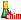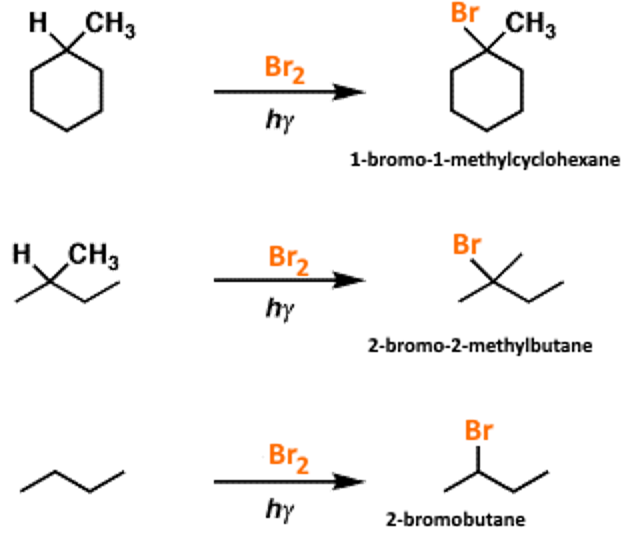﻿ Reactions between alkanes and halogensSearch:

# Reactions between alkanes and halogens

## Fluorine

Fluorine destroys alkanes to form carbon and hydrogen fluoride, for example: $C_5H_{10}+ 5F_2 \; \rightarrow \; 5C + 10HF$ The reaction is often explosive.

## Chlorine

Chlorine in the presence of light replaces hydrogen atoms of alkanes to form chloralkanes and hydrogène chloride, for example: $C_2H_6 + Cl_2 \; \rightarrow \; HCl + C_2H_5Cl$ - In this reaction, the secondary or tertiary $H$ atoms are favored, for example, in the chlorination of butane: (1) $CH_3CH_2CH_2CH_3 + Cl_2$ $\rightarrow$ $CH_3CH_2CH_2CH_2Cl + HCl$ or (2) $CH_3CH_2CH_2CH_3 + Cl_2$ $\rightarrow$ $CH_3CH_2CHClCH_3 + HCl$ The proportion 1-chlorobutane: 2-chlorobutane should be $6 : 4$ since the butane has 6 chain end $H$ atoms and only 4 in the middle, whereas in reality this proportion is $29: 71$ - Excess chlorine leads to polysubstitutions, for example: Chloromethane formation: $CH_4 + Cl_2 \; \rightarrow \; HCl + CH_3Cl$ Dichloromethane formation: $CH_4 + Cl_2 \; \rightarrow \; HCl + CH_3Cl$ Trichloromethane formation: $CH_2Cl_2 + Cl_2\; \rightarrow \; HCl + CHCl_3$ Tetrachloromethane formation: $CHCl_3 + Cl_2\; \rightarrow \; HCl + CCl_4$

## Bromine

Bromine in the presence of light replaces hydrogen atoms of alkanes to form bromalkanes and bromide hyydrogène, for example:$C_2H_6 + Br_2 \; \rightarrow \; C_2H_5Br + HBr$ Bromine is brown, bromohexane is colorless and hydrogen bromide also, where the observed discoloration. The shift of litmus paper from blue to red reveals the production of gaseous hydrogen bromide. - You will find the video of this experience →       here - In this reaction, the secondary or tertiary carbon $H$ are strongly favored. Here are, for example, the bromination products which are largely in the majority in the following reactions:## Iodine

Iodine even in the presence of light will not replace the hydrogen atoms of alkanes.